Comparing rational numbers

Chapter 8 Class 7 Rational Numbers
Concept wise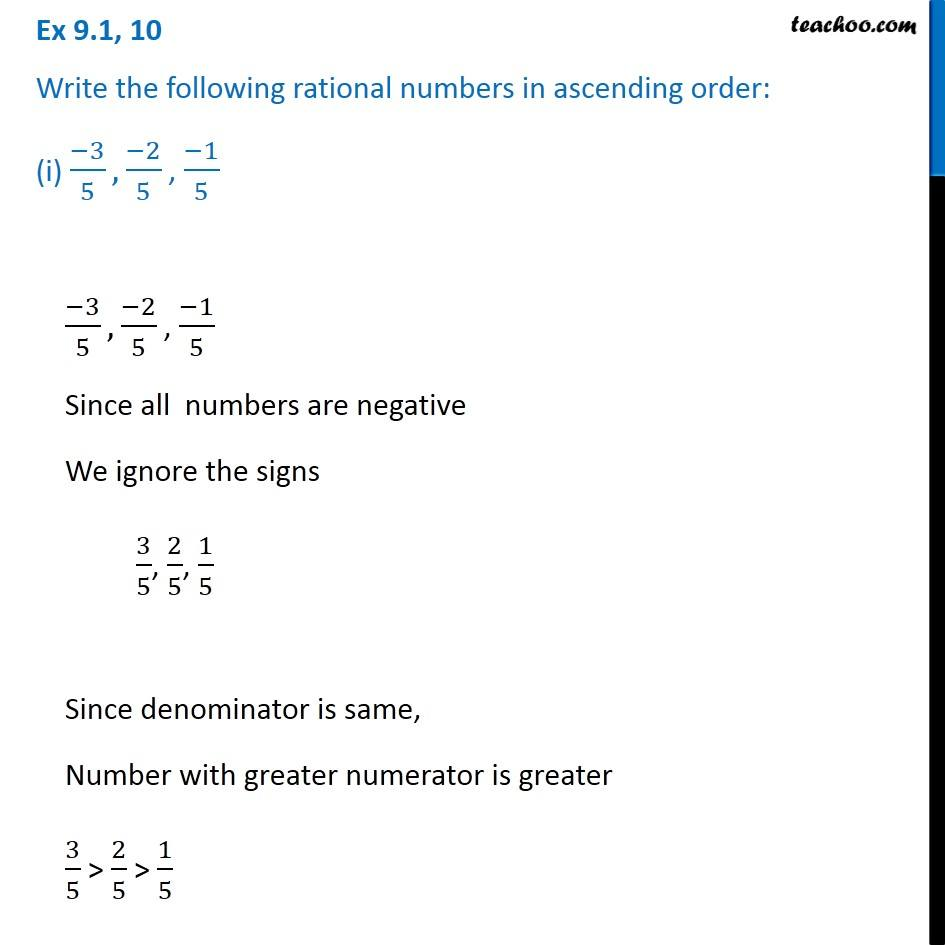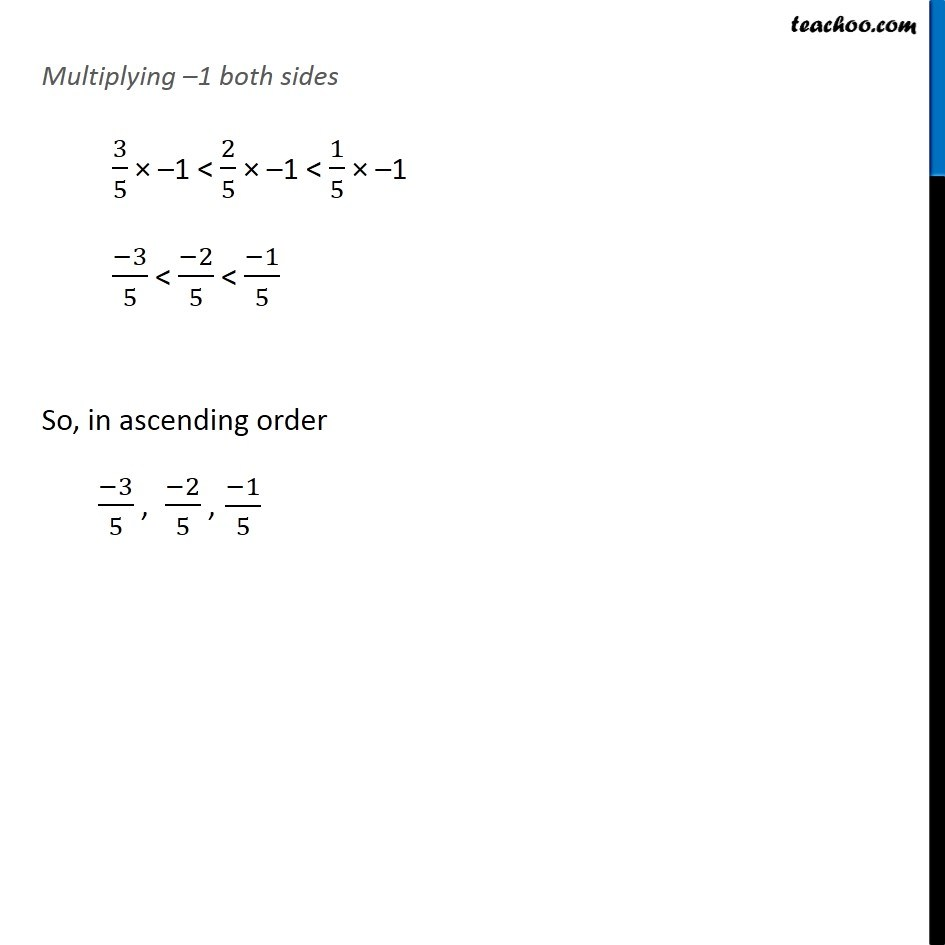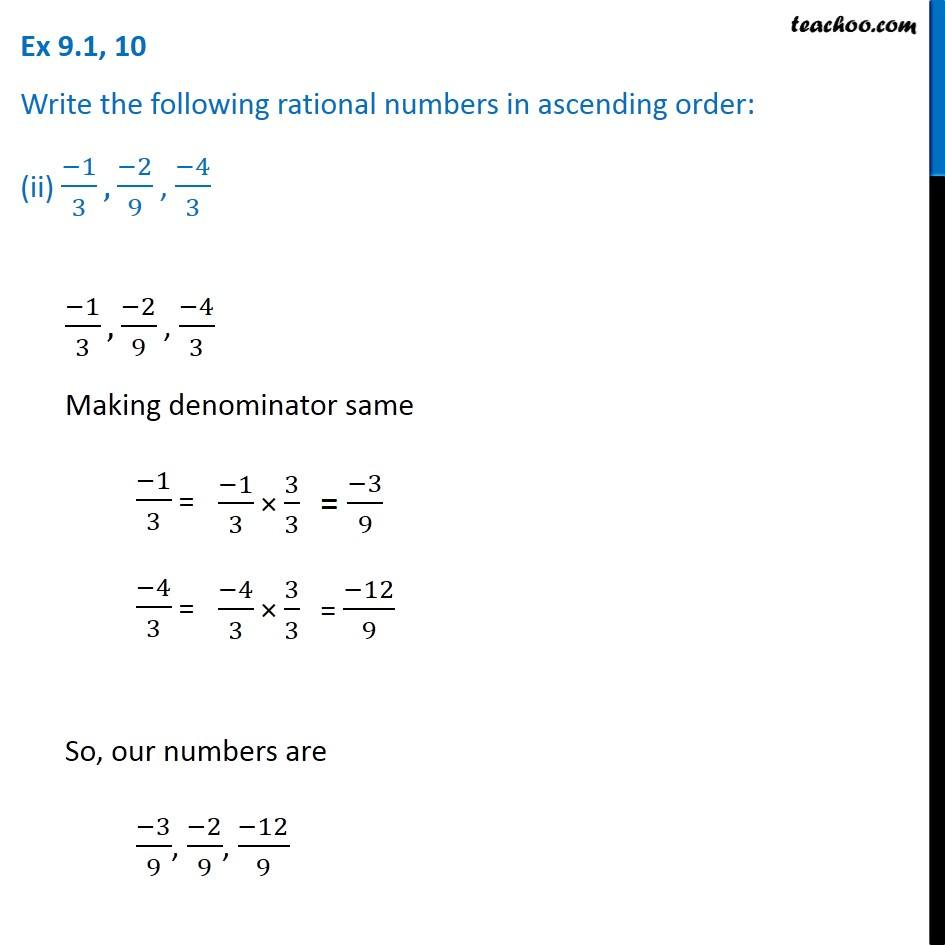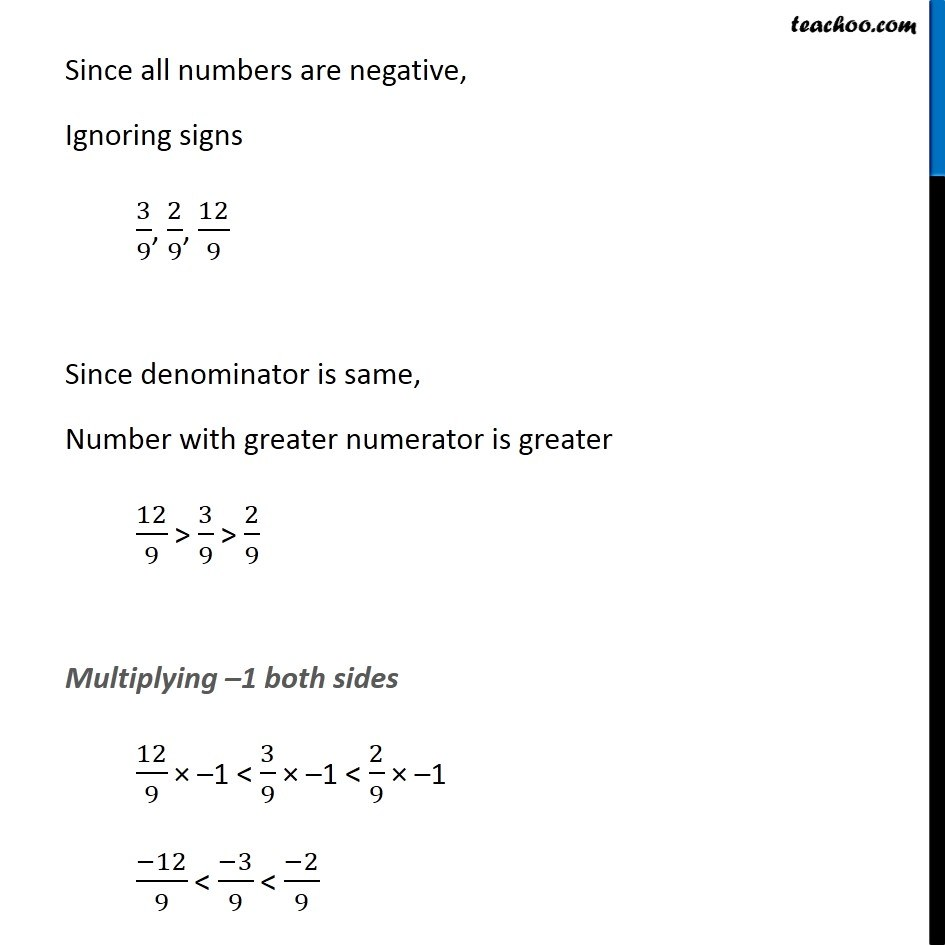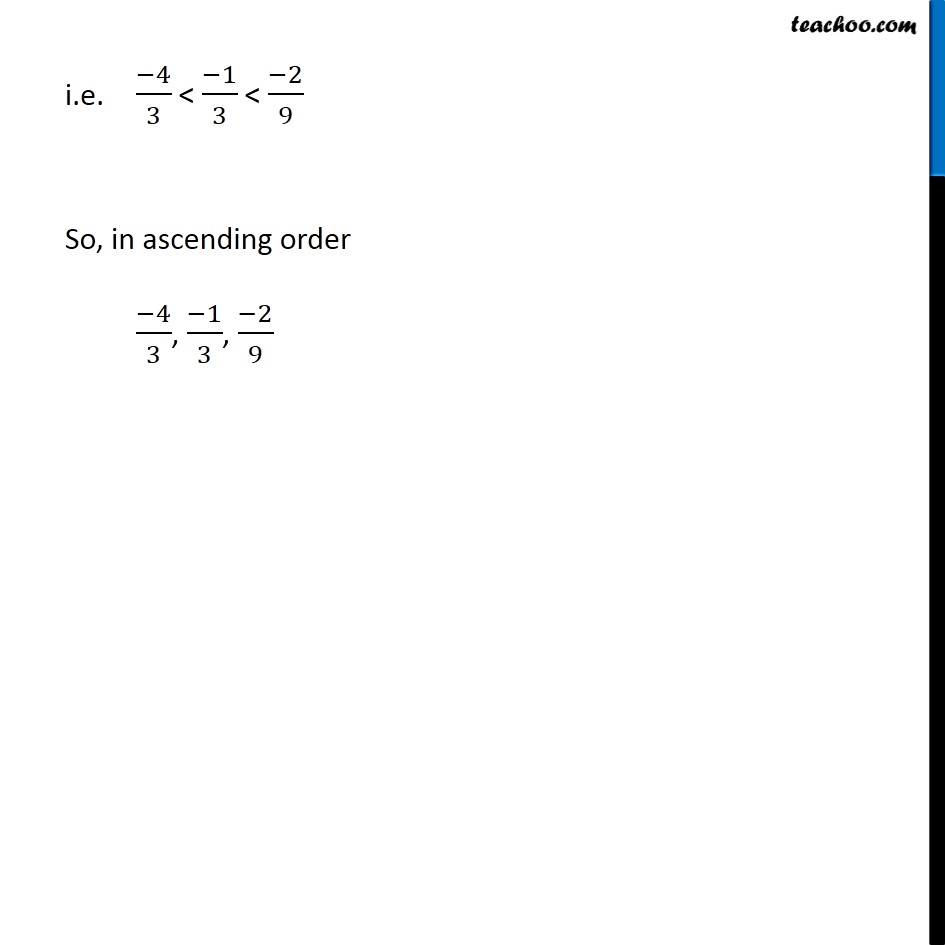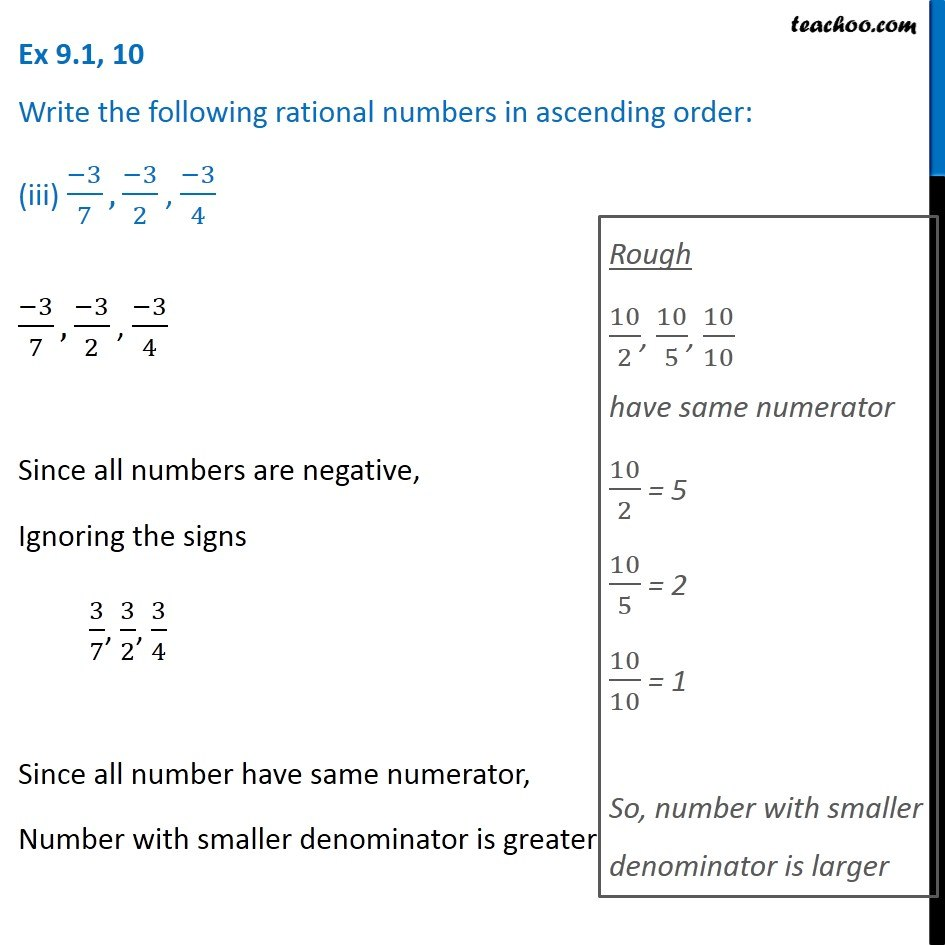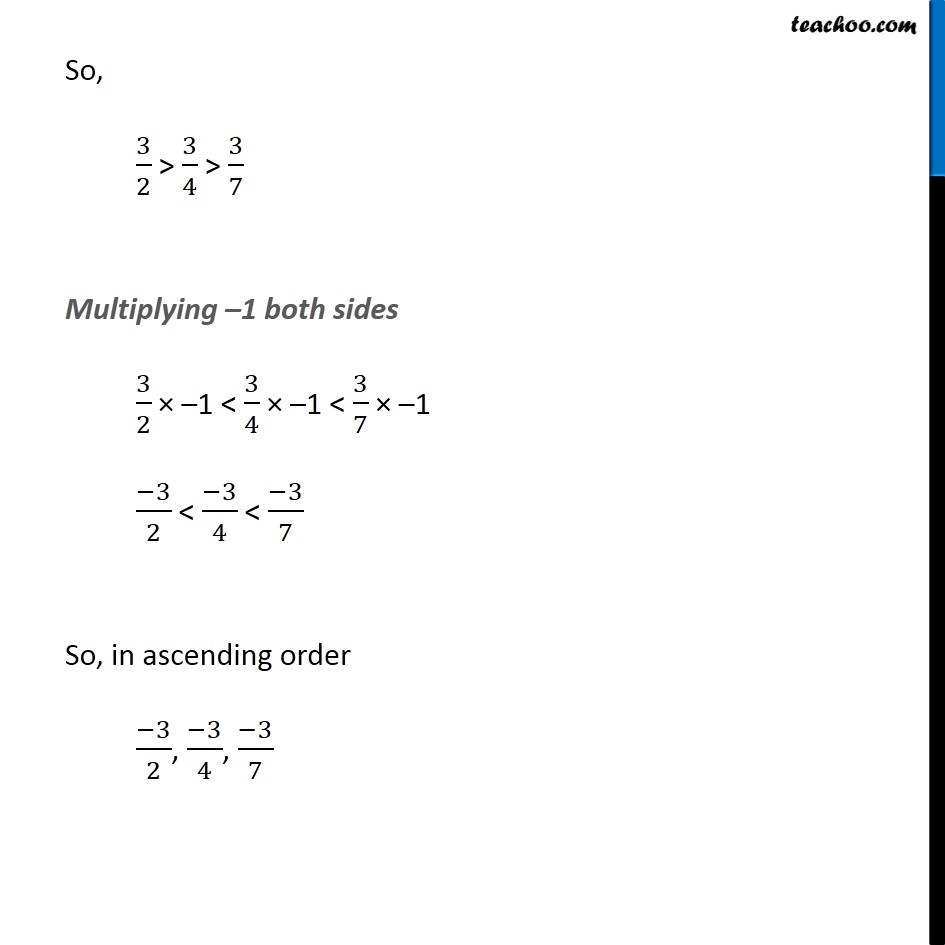Learn in your speed, with individual attention - Teachoo Maths 1-on-1 Class

### Transcript

Ex 8.1, 10 Write the following rational numbers in ascending order: (i) (−3)/5, (−2)/5 , (−1)/5 (−3)/5, (−2)/5 , (−1)/5 Since all numbers are negative We ignore the signs 3/5, 2/5, 1/5 Since denominator is same, Number with greater numerator is greater 3/5 > 2/5 > 1/5 Multiplying –1 both sides 3/5 × –1 < 2/5 × –1 < 1/5 × –1 (−3)/5 < (−2)/5 < (−1)/5 So, in ascending order (−3)/5 ,(−2)/5 ,(−1)/5 Ex 8.1, 10 Write the following rational numbers in ascending order: (ii) (−1)/3, (−2)/9 , (−4)/3 (−1)/3, (−2)/9 , (−4)/3 Making denominator same (−1)/3 = (−4)/3 = So, our numbers are (−3)/9, (−2)/9, (−12)/9 Since all numbers are negative, Ignoring signs 3/9, 2/9, 12/9 Since denominator is same, Number with greater numerator is greater 12/9 > 3/9 > 2/9 Multiplying –1 both sides 12/9 × –1 < 3/9 × –1 < 2/9 × –1 (−12)/9 < (−3)/9 < (−2)/9 i.e. (−4)/3 < (−1)/3 < (−2)/9 So, in ascending order (−4)/3, (−1)/3, (−2)/9 Ex 8.1, 10 Write the following rational numbers in ascending order: (iii) (−3)/7, (−3)/2 , (−3)/4 (−3)/7, (−3)/2 , (−3)/4 Since all numbers are negative, Ignoring the signs 3/7, 3/2, 3/4 Since all number have same numerator, Number with smaller denominator is greater Rough 10/2, 10/5, 10/10 have same numerator 10/2 = 5 10/5 = 2 10/10 = 1 So, number with smaller denominator is larger So, 3/2 > 3/4 > 3/7 Multiplying –1 both sides 3/2 × –1 < 3/4 × –1 < 3/7 × –1 (−3)/2 < (−3)/4 < (−3)/7 So, in ascending order (−3)/2, (−3)/4, (−3)/7